# RFLPtools: Analysis of DNA fragment samples and standalone BLAST report files

## Introduction

Package RFLPtools aims at

• the detection of similar band patterns based on DNA fingerprint fragment sizes (i.e. derived from RFLP-analysis)

• the analysis of standalone BLAST report files (i.e. DNA sequence analysis)

see also (Flessa, Kehl, and Kohl 2013).

In this short vignette we describe and demonstrate the available functions.

We start with loading the package.

library(RFLPtools)
## Loading required package: RColorBrewer

## RFLP data

As a first step we can perform a QC of the samples using function RFLPqc. This is possible, if the first band corresponds to the total length of the fragment. The QC consists of a comparison of the length of the first band with the sum of the lengths of the remaining bands for each sample. If the sum is smaller than QC.lo times the length of the first band or larger than QC.up times the length of the first band, respectively, a text message is printed.

Dir <- system.file("extdata", package = "RFLPtools") # input directory
filename <- file.path(Dir, "AZ091016_report.txt")
RFLP1 <- read.rflp(file = filename)
str(RFLP1)
## 'data.frame':    73 obs. of  4 variables:
##  $Sample: chr "AZ38_B1" "AZ38_B1" "AZ38_B1" "AZ38_C1" ... ##$ Band  : int  1 2 3 1 2 3 1 2 3 1 ...
##  $MW : int 767 443 257 763 439 254 760 459 238 750 ... ##$ Gel   : chr  "091016A" "091016A" "091016A" "091016A" ...
RFLP2 <- RFLPqc(RFLP1, rm.band1 = FALSE) # identical to RFLP1
## Sum of bands of sample AZ38_B2 out of range (227.97%)!
## Sum of bands of sample AZ38_C2 out of range (69.47%)!
identical(RFLP1, RFLP2)
##  TRUE
RFLP3 <- RFLPqc(RFLP1)
## Sum of bands of sample AZ38_B2 out of range (227.97%)!
## Sum of bands of sample AZ38_C2 out of range (69.47%)!
str(RFLP3)
## 'data.frame':    51 obs. of  4 variables:
##  $Sample: chr "AZ38_B1" "AZ38_B1" "AZ38_C1" "AZ38_C1" ... ##$ Band  : num  1 2 1 2 1 2 1 2 3 4 ...
##  $MW : int 443 257 439 254 459 238 212 186 144 97 ... ##$ Gel   : chr  "091016A" "091016A" "091016A" "091016A" ...
RFLP4 <- RFLPqc(RFLP1, rm.band1 = TRUE, QC.rm = TRUE)
## Sum of bands of sample AZ38_B2 out of range (227.97%)!
## Sum of bands of sample AZ38_C2 out of range (69.47%)!
str(RFLP4)
## 'data.frame':    43 obs. of  4 variables:
##  $Sample: chr "AZ38_B1" "AZ38_B1" "AZ38_C1" "AZ38_C1" ... ##$ Band  : num  1 2 1 2 1 2 1 2 3 4 ...
##  $MW : int 443 257 439 254 459 238 212 186 144 97 ... ##$ Gel   : chr  "091016A" "091016A" "091016A" "091016A" ...

We load some example data and compute the Euclidean distance …

data(RFLPdata)
res <- RFLPdist(RFLPdata)
names(res) ## number of bands
##   "3"  "4"  "5"  "6"  "7"  "8"  "9"  "10" "11" "12"
str(res$"6") ## 'dist' num [1:210] 517.58 3.74 145.24 397.64 482.89 ... ## - attr(*, "Size")= int 21 ## - attr(*, "Labels")= chr [1:21] "Ni_25_A3" "Ni_25_B1" "Ni_25_B3" "Ni_25_H5" ... ## - attr(*, "Diag")= logi FALSE ## - attr(*, "Upper")= logi FALSE ## - attr(*, "method")= chr "euclidean" ## - attr(*, "call")= language distfun(x = do.call("rbind", temp1)) Of course, we can also use other well-known distances implemented in function dist. res1 <- RFLPdist(RFLPdata, distfun = function(x) dist(x, method = "manhattan")) res2 <- RFLPdist(RFLPdata, distfun = function(x) dist(x, method = "maximum")) str(res[]) ## 'dist' num [1:28] 2.45 18.25 12.96 155.64 4.9 ... ## - attr(*, "Size")= int 8 ## - attr(*, "Labels")= chr [1:8] "NI_28_D6" "Ni_28_A6" "Ni_28_B2" "Ni_28_C2" ... ## - attr(*, "Diag")= logi FALSE ## - attr(*, "Upper")= logi FALSE ## - attr(*, "method")= chr "euclidean" ## - attr(*, "call")= language distfun(x = do.call("rbind", temp1)) str(res1[]) ## 'dist' num [1:28] 4 31 20 209 8 21 176 27 16 211 ... ## - attr(*, "Size")= int 8 ## - attr(*, "Labels")= chr [1:8] "NI_28_D6" "Ni_28_A6" "Ni_28_B2" "Ni_28_C2" ... ## - attr(*, "Diag")= logi FALSE ## - attr(*, "Upper")= logi FALSE ## - attr(*, "method")= chr "manhattan" ## - attr(*, "call")= language dist(x = x, method = "manhattan") str(res2[]) ## 'dist' num [1:28] 2 13 10 146 4 8 104 12 9 147 ... ## - attr(*, "Size")= int 8 ## - attr(*, "Labels")= chr [1:8] "NI_28_D6" "Ni_28_A6" "Ni_28_B2" "Ni_28_C2" ... ## - attr(*, "Diag")= logi FALSE ## - attr(*, "Upper")= logi FALSE ## - attr(*, "method")= chr "maximum" ## - attr(*, "call")= language dist(x = x, method = "maximum") Correlation distances can for instance be applied by using function corDist of package MKomics. library(MKomics) res3 <- RFLPdist(RFLPdata, distfun = corDist) str(res3$"9")
##  'dist' num [1:21] 0.475 0.521 0.508 0.517 0.512 ...
##  - attr(*, "Size")= int 7
##  - attr(*, "Labels")= chr [1:7] "Ni_25_C4" "Ni_25_C5" "Ni_25_E4" "Ni_28_B9" ...
##  - attr(*, "Diag")= logi FALSE
##  - attr(*, "Upper")= logi FALSE
##  - attr(*, "method")= chr "pearson"
##  - attr(*, "call")= language distfun(x = do.call("rbind", temp1))

As we obtain a list of dist objects we can easily perform hierarchical clustering.

plot(hclust(res[]), main = "Euclidean distance")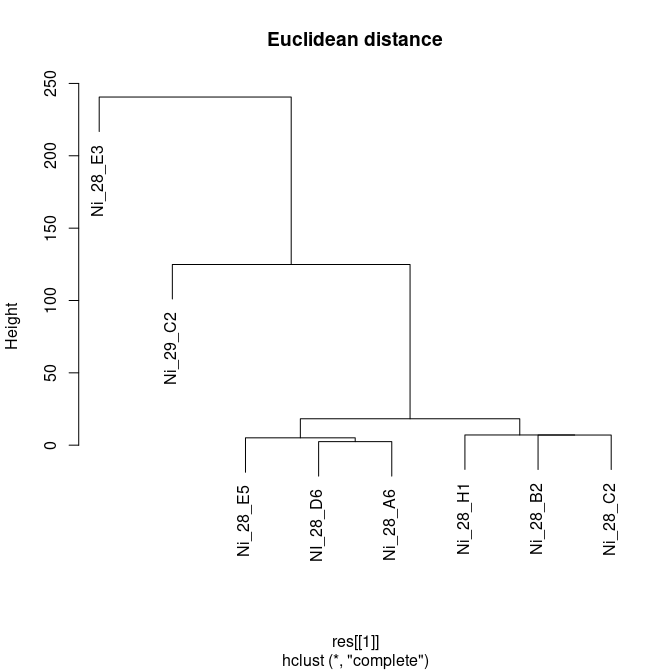plot(hclust(res1[]), main = "Manhattan distance")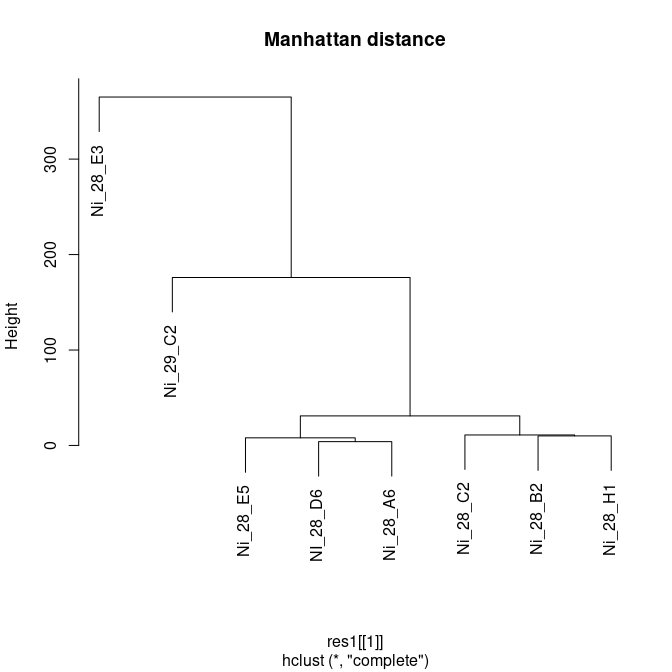plot(hclust(res2[]), main = "Maximum distance")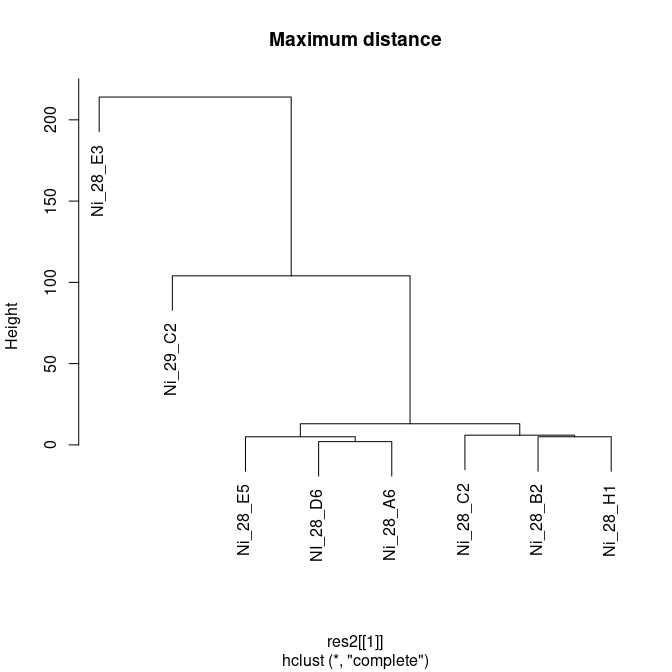plot(hclust(res3[]), main = "Pearson correlation distance")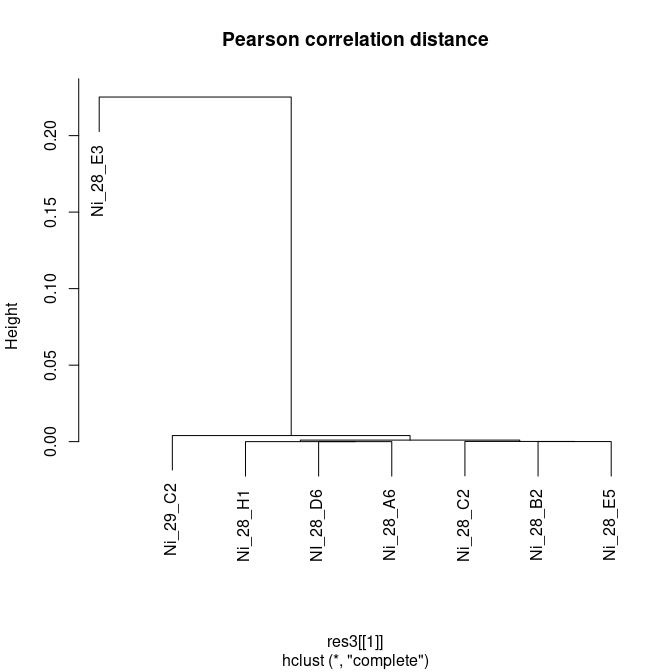For splitting the dendrogram into clusters we apply function cutree.

clust4bd <- hclust(res[])
cgroups50 <- cutree(clust4bd, h=50)
cgroups50
## Ni_25_B2 Ni_25_B5 Ni_28_A2 Ni_28_A9 Ni_28_B4 Ni_28_B5 Ni_28_D4 Ni_28_E1
##        1        2        3        4        5        3        3        6
## Ni_28_E4 Ni_28_F2 Ni_28_F4 Ni_28_G4 Ni_28_H4 Ni_29_A3 Ni_29_A4 Ni_29_A7
##        3        3        3        3        3        7        8        8
## Ni_29_B4 Ni_29_B5 Ni_29_C7 Ni_29_D1 Ni_29_D6 Ni_29_D7 Ni_29_E4 Ni_29_E5
##        9       10       11       12       13       14        8        9
## Ni_29_F5 Ni_29_G1 Ni_29_G2 Ni_29_G4 Ni_29_H2 Ni_29_H4 Ni_29_H5
##       11        7       11       15        7        8       13

Another possibility to display the similarity of the samples are so-called (dis-)similarity matrices that can for instance be generated by function simPlot of package .

library(RColorBrewer)
library(MKomics)
myCol <- colorRampPalette(brewer.pal(8, "RdYlGn"))(128)
ord <- order.dendrogram(as.dendrogram(hclust(res[])))
temp <- as.matrix(res[])
simPlot(temp[ord,ord], col = rev(myCol), minVal = 0,
labels = colnames(temp), title = "(Dis-)Similarity Plot")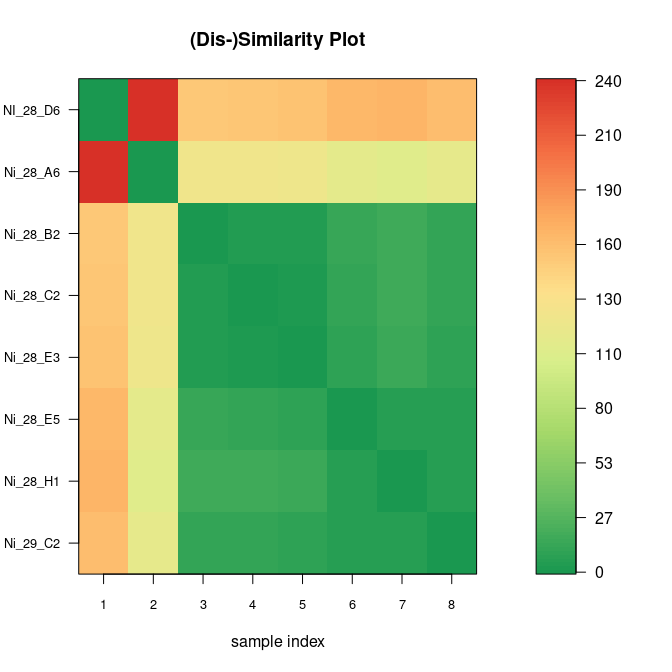We can also use function levelplot of package lattice to display (dis-)similarity matrices.

library(lattice)
print(levelplot(temp[ord,ord], col.regions = rev(myCol),
at = do.breaks(c(0, max(temp)), 128),
xlab = "", ylab = "",
## Rotate labels of x-axis
scales = list(x = list(rot = 90)),
main = "(Dis-)Similarity Plot"))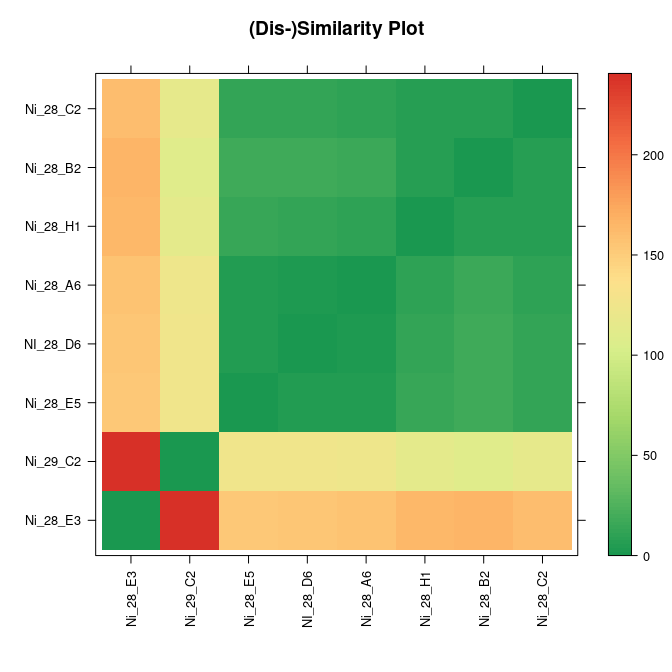If some bands may be missing, we can apply function RFLPdist2 specifying the number of missing bands we expect.

## Euclidean distance
data(RFLPdata)
data(RFLPref)
nrBands(RFLPdata)
##    3  4  5  6  7  8  9 10 11 12
res0 <- RFLPdist(RFLPdata, nrBands = 9)
res1 <- RFLPdist2(RFLPdata, nrBands = 9, nrMissing = 1)
res2 <- RFLPdist2(RFLPdata, nrBands = 9, nrMissing = 2)
res3 <- RFLPdist2(RFLPdata, nrBands = 9, nrMissing = 3)

Again hierarchical clustering of the results is straight forward.

plot(hclust(res0), main = "0 bands missing")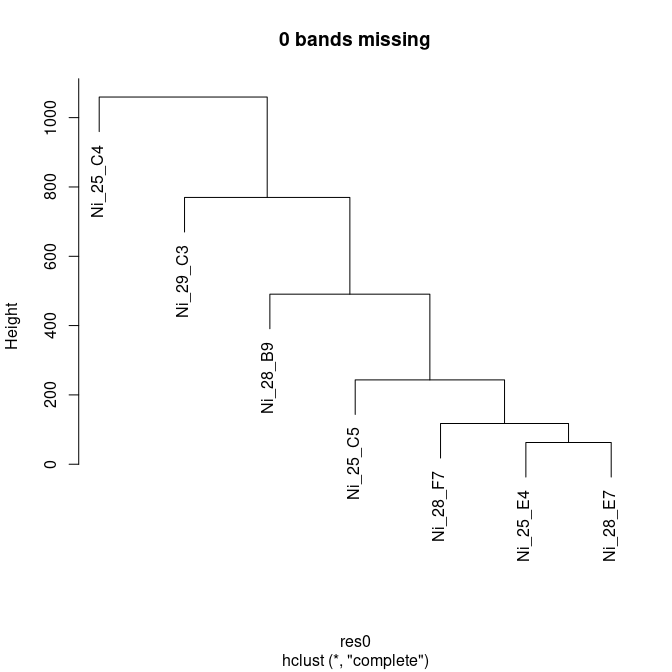plot(hclust(res1), main = "1 band missing")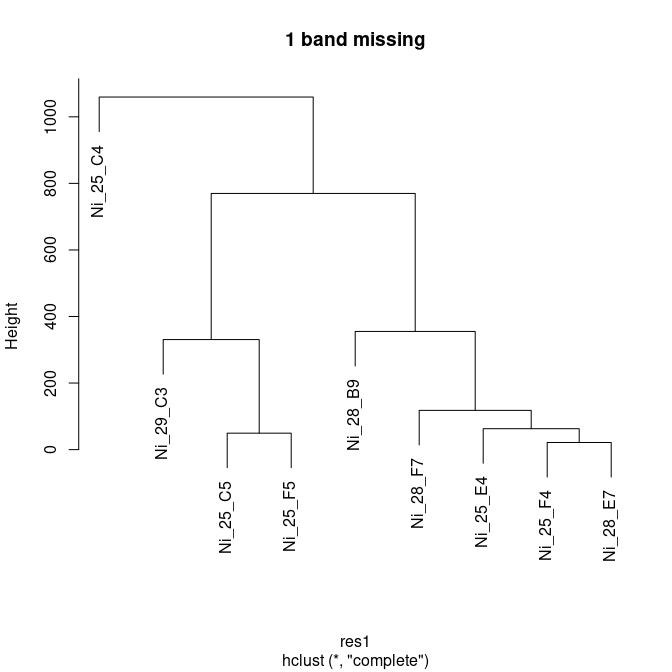plot(hclust(res2), main = "2 bands missing")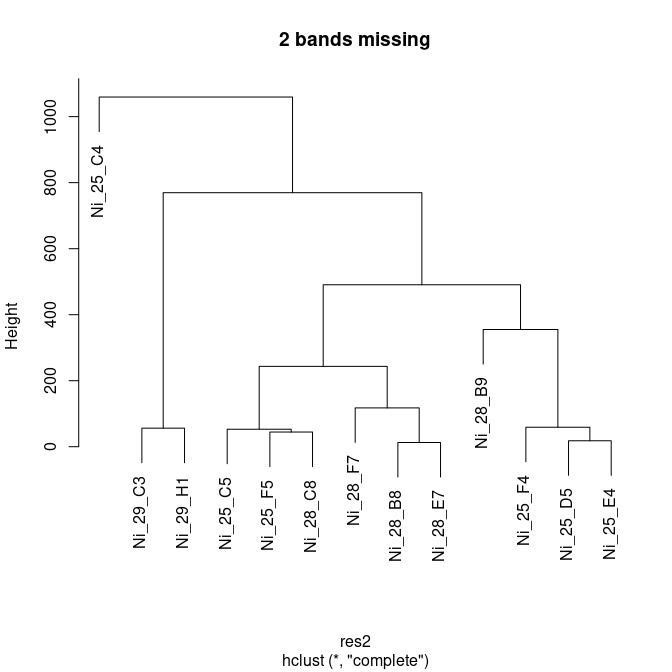plot(hclust(res3), main = "3 bands missing")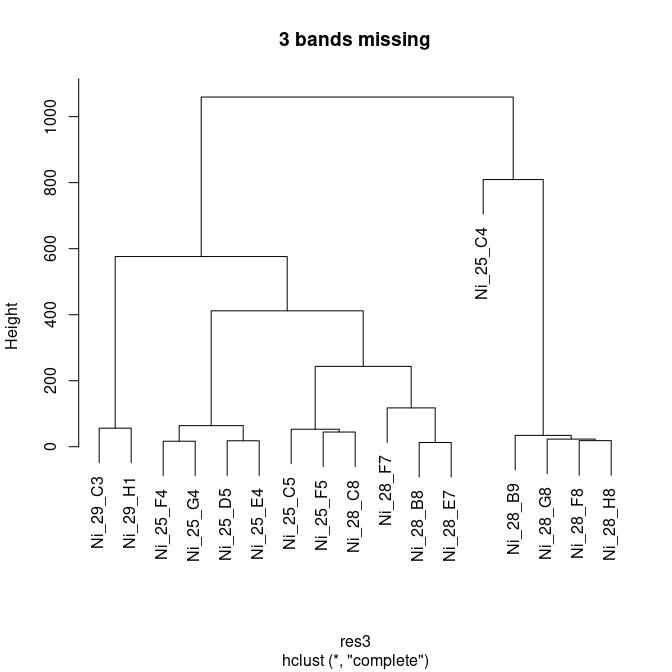There are also ways to handle low-bp bands, which are likely to be absent in some of the samples. First, one can use function RFLPlod to remove all bands below a given threshold LOD before further analyses. For displaying the data we use function RFLPplot.

RFLPdata.lod <- RFLPlod(RFLPdata, LOD = 60)
## 20 bands were removed.
par(mfrow = c(1, 2))
RFLPplot(RFLPdata, nrBands = 4, ylim = c(40, 670))
RFLPplot(RFLPdata.lod, nrBands = 4, ylim = c(40, 670))
title(sub = "After applying RFLPlod")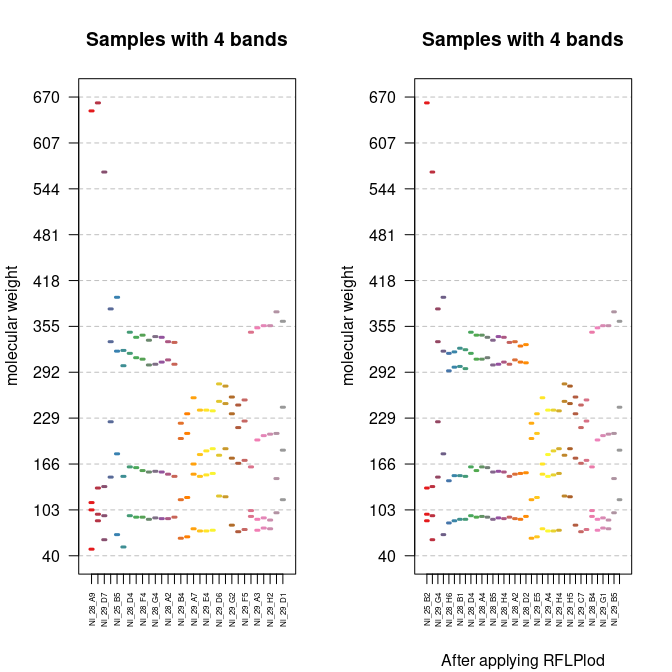In addition, one can specify LOD in a call to function RFLPdist2. If LOD is specified, it is assumed that missing bands only occur for molecular weights smaller than LOD.

res0 <- RFLPdist(RFLPdata, nrBands = 4)
res1.lod <- RFLPdist2(RFLPdata, nrBands = 4, nrMissing = 1, LOD = 60)
ord <- order.dendrogram(as.dendrogram(hclust(res1.lod)))
temp <- as.matrix(res1.lod)
simPlot(temp[ord,ord], col = rev(myCol), minVal = 0,
labels = colnames(temp),
title = "(Dis-)Similarity Plot\n1 band missing below LOD")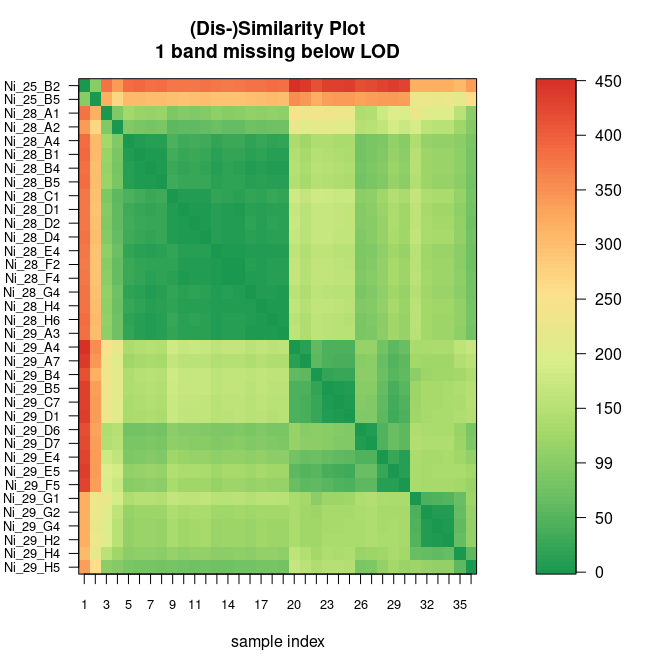We can also make a comparison to reference data.

RFLPrefplot(RFLPdata, RFLPref, nrBands = 9, cex.axis = 0.8)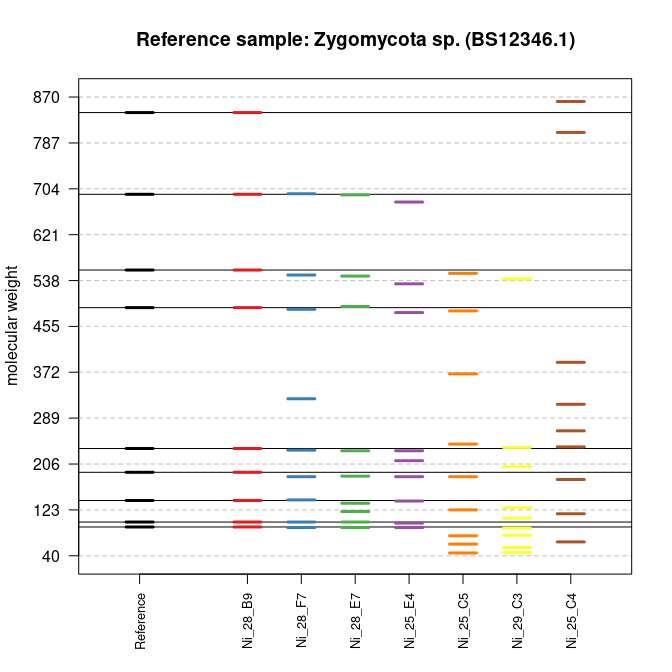## BLAST data

To analyze tabular report files generated with standalone BLAST from NCBI (see https://blast.ncbi.nlm.nih.gov/Blast.cgi?CMD=Web&PAGE_TYPE=BlastDocs&DOC_TYPE=Download), a function for reading the BLAST report files is included (read.blast).

Possible steps are:

1. Install NCBI BLAST
2. Generate and import database(s)
3. Apply BLAST with options outfmt and out; e.g.

blastn -query Testquery -db Testdatabase -outfmt 6 -out out.txt

or

blastn -query Testquery -db Testdatabase -outfmt 10 -out out.csv

One could also call BLAST from inside R by using function system

system("blastn -query Testquery -db Testdatabase -outfmt 6 -out out.txt")
1. Read in the results
## -outfmt 6
test.res <- read.blast(file = "out.txt")

or

## -outfmt 10
test.res <- read.blast(file = "out.csv", sep = ",")

We now read in a example file included in folder extdata of our package.

Dir <- system.file("extdata", package = "RFLPtools") # input directory
filename <- file.path(Dir, "BLASTexample.txt")
BLAST1 <- read.blast(file = filename)
str(BLAST1)
## 'data.frame':    4069 obs. of  12 variables:
##  $query.id : chr "agrFF002" "agrFF002" "agrFF002" "agrFF002" ... ##$ subject.id      : chr  "agrFF002" "agrFF148" "agrFF148" "agrFF176" ...
##  $identity : num 100 93.4 100 91.4 100 ... ##$ alignment.length: int  544 243 11 255 11 255 11 256 11 256 ...
##  $mismatches : int 0 14 0 20 0 20 0 18 0 18 ... ##$ gap.opens       : int  0 2 0 2 0 2 0 3 0 3 ...
##  $q.start : int 1 199 462 187 462 187 462 187 462 187 ... ##$ q.end           : int  544 439 472 439 472 439 472 439 472 439 ...
##  $s.start : int 1 671 785 123 250 121 248 121 248 126 ... ##$ s.end           : int  544 913 795 377 260 375 258 375 258 380 ...
##  $evalue : num 0.0 6.0e-102 6.7 2.0e-100 6.7 ... ##$ bit.score       : num  944 360 21.1 354 21.1 354 21.1 352 21.1 352 ...

This example BLAST data is also available as loadable example data.

data(BLASTdata)

The loaded data.frame can be used to compute similarities between the BLASTed sequences via function simMatrix. This function includes the following steps:

1. the length of each sequence (LS) comprised in the input data file is extracted.

2. if there is more than one comparison for one sequence including different parts of the respective sequence, that one with maximum base length is chosen.

3. the number of matching bases (mB) is calculated by multiplying two variables given in the BLAST output: the identity between sequences (%) and the number of nucleotides divided by 100.

4. the resulting value is rounded to the next integer.

5. the similarity is calculated by dividing mB by LS and saved in the corresponding similarity matrix.

If the similarity of a combination is not shown in the BLAST report file (because the similarity was lower than 70%), this comparison is included in the similarity matrix with the result zero.

res <- simMatrix(BLASTdata)

Optionally, the range of sequence length can be specified to exclude sequences, which were too short or too long, respectively.

res1 <- simMatrix(BLASTdata, sequence.range = TRUE, Min = 100, Max = 450)
res2 <- simMatrix(BLASTdata, sequence.range = TRUE, Min = 500)

We display the similarity matrix.

library(MKomics)
simPlot(res2, col = myCol, minVal = 0, cex.axis = 0.5,
labels = colnames(res2), title = "(Dis-)Similarity Plot")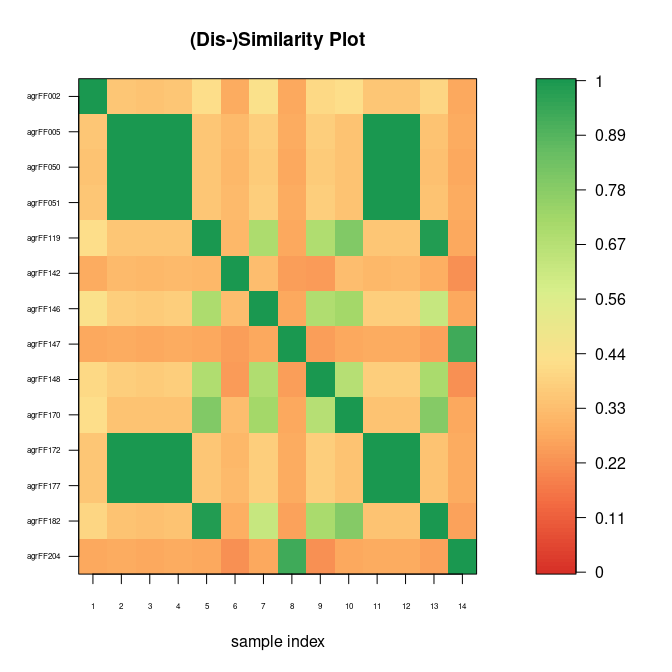Alternatively, we can again use function levelplot of package lattice.

library(lattice)
txt <- trellis.par.get("add.text")
txt\$cex <- 0.5
trellis.par.set("add.text" = txt)
myCol <- colorRampPalette(brewer.pal(8, "RdYlGn"))(128)
print(levelplot(res2, col.regions = myCol,
at = do.breaks(c(0, max(res2)), 128),
xlab = "", ylab = "",
## Rotate labels of x axis
scales = list(x = list(rot = 90)),
main = "(Dis-)Similarity Plot"))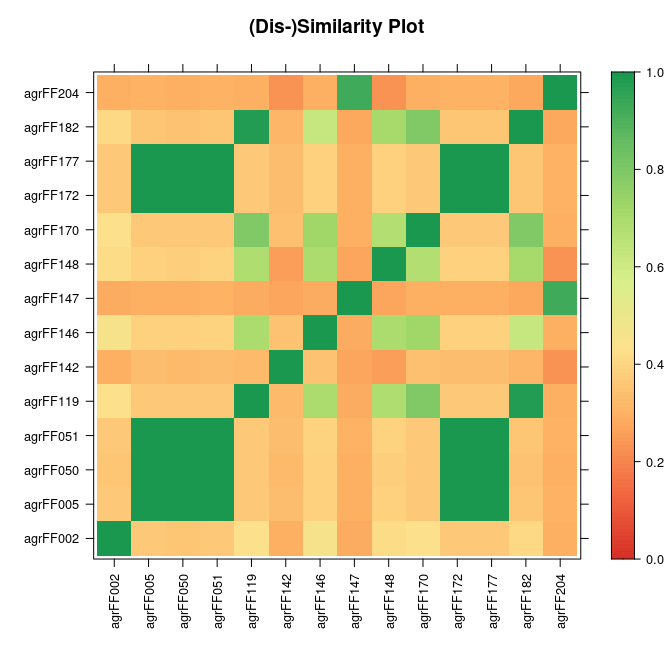We can also convert the similarity matrix to an object of S3 class “dist”.

res.d <- sim2dist(res2)

After the conversion we can for instance perform hierarchical clustering.

## hierarchical clustering
plot(hclust(res.d), cex = 0.7)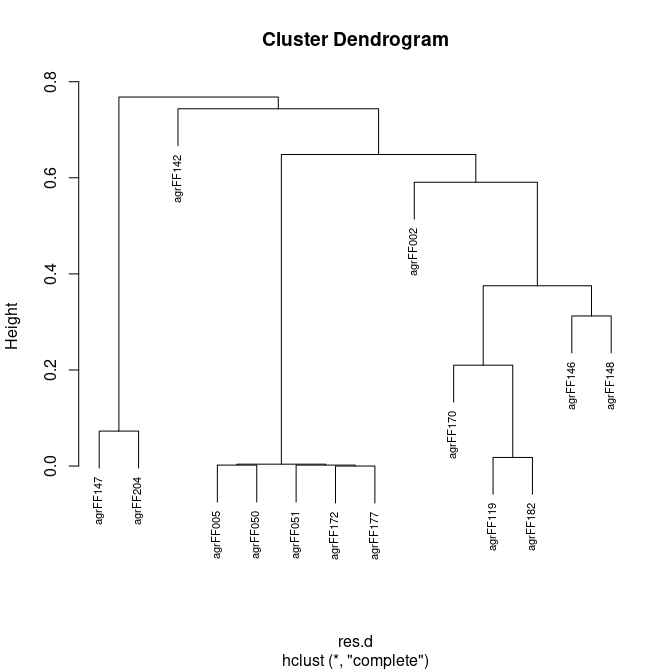## sessionInfo

sessionInfo()
## R version 4.0.4 Patched (2021-02-17 r80031)
## Platform: x86_64-pc-linux-gnu (64-bit)
## Running under: Linux Mint 20.1
##
## Matrix products: default
## BLAS:   /usr/lib/x86_64-linux-gnu/libf77blas.so.3.10.3
## LAPACK: /home/kohlm/RTOP/Rbranch/lib/libRlapack.so
##
## locale:
##   LC_CTYPE=de_DE.UTF-8       LC_NUMERIC=C
##   LC_TIME=de_DE.UTF-8        LC_COLLATE=C
##   LC_MONETARY=de_DE.UTF-8    LC_MESSAGES=de_DE.UTF-8
##   LC_PAPER=de_DE.UTF-8       LC_NAME=C
##   LC_ADDRESS=C               LC_TELEPHONE=C
##  LC_MEASUREMENT=de_DE.UTF-8 LC_IDENTIFICATION=C
##
## attached base packages:
##  stats     graphics  grDevices utils     datasets  methods   base
##
## other attached packages:
##  lattice_0.20-41    MKomics_0.6        RFLPtools_1.8      RColorBrewer_1.1-2
##
## loaded via a namespace (and not attached):
##   digest_0.6.27     grid_4.0.4        magrittr_2.0.1    evaluate_0.14
##   rlang_0.4.10      stringi_1.5.3     limma_3.44.3      robustbase_0.93-7
##   rmarkdown_2.6     tools_4.0.4       stringr_1.4.0     DEoptimR_1.0-8
##  xfun_0.20         yaml_2.2.1        compiler_4.0.4    htmltools_0.5.0
##  knitr_1.30